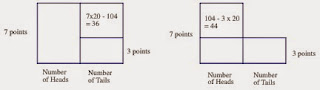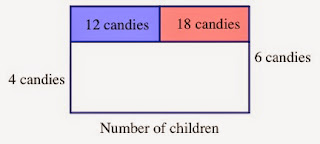## Tuesday, November 18, 2014

### Area model as a reasoning tool (2)

In the last entry, which was posted more than 3 months ago, I discussed how area models might be used to make sense of and solve some challenging mathematics problems. I ended the entry with 2 problems for you to solve using area models.

Suppose there is a simple coin-toss game. On each toss, you receive 7 points if you get a Heads and 3 points if you get a Tails. After you played the game 20 times (that is, 20 coin tosses), your score was 104 points. How many times did you get Heads? How many times did you get Tails?

In this problem the points you earn is the sum of the points you earn by getting Heads and the points you earn by getting Tails. The point you earn by getting Heads is the product of 7 and the number of Heads, and the point you earn by getting Tails is the product of 3 and the number of Tails. Since a product can be represented by an area model, you can draw the following area model to represent this situation.Since the total number of tosses is 20, (Number of Heads) + (Number of Tails) = 20. Moreover, the total points earned was 104 points, so the total area must be 104. So, this is just like the chicken and pig problem. So, by using the same reasoning we discussed last time, you can use one of the following approaches.From the picture on the left we can see that (Number of Tails) × 4 = 36. So, the number of tails must have been 9, thus the number of heads was 11. Or, from the picture on the right, we can see that (Number of Heads) × 4 = 44, and we can get the number of heads to be 11.

The second problem was a bit different.

There are some candies and children. If we give each child 4 candies there will be 18 candies left. However, in order to give each child 6 candies, we will need 12 additional candies. How many candies and how many children are there?

The number of candies must be somewhere between (Number of children) × 4 and (Number of children) × 6. If we represent the number of children as the horizontal side of the area model, then the two rectangles that represent these two products will have the same "width" but the different height.So, we can represent the total number of candies by a L-shape like this:Moreover, since we know that there will be 18 candies left when we give each child 4 candies, we can show that fact like this.Finally, since we know that we will be short 12 candies if we try to give each child 6 candies, we can represent that fact like this.Since the vertical dimension of the two colored rectangles is 2, that is, the difference between 6 candies and 4 candies, we can easily tell that there were 15 children. The total number of candies can be calculated either 4 × 15 + 18 = 78 or 6 × 15 − 12 = 78.

I hope these two entries piqued your interest in area model as a reasoning tool. I encourage you to try to find other problems that can be represented and solved arithmetically using this tool.